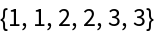Function Repository Resource:

# DihedralODE

Find the ordinary differential equation constraining periods of certain symmetrical curves

 ResourceFunction["DihedralODE"][H,{λ,ϕ,α}] returns the ODE constraining integral periods T(α), given a Hamiltonian H such that α=H(λ,cos(k ϕ)). ResourceFunction["DihedralODE"][H,{λ,ϕ,α},rules] first applies coordinate rewrite rules to H. ResourceFunction["DihedralODE"][H,"Certificate"] assumes default coordinate handling and returns a checked and checkable Association containing proof data.

## Details

The acronym ODE stands for "ordinary differential equation."
Specification of variables {λ,ϕ,α} is optional if the Hamiltonian is written in terms of the formal action-angle variables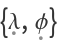.
Input Hamiltonian H should be polynomial in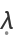and linear in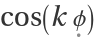, something like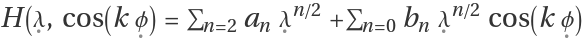.
Input Hamiltonian H can also be specified in Cartesian (p,q) or spherical (X,Y,Z) coordinates.
Spherical curves are drawn on the surface of a unit sphere, X 2+Y 2+Z 2=1.
The default rewrite rules change from either system to action-angle coordinates:
 Cartesian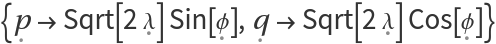Spherical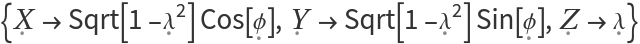Note: Both the plane and the sphere are symplectic manifolds with canonical action-angle coordinates.
Time t is defined in terms of the tangents Dt{λ,ϕ}={-ϕH,λH}.
The invariant time differential may then be written most directly as dt=/(λH).
Subsequent α-derivatives of the integrand dt are written as (α)ndt=(λH)dt(α)n1/(λH).
Here we operate α through λ via the chain rule with αλ=1/(λH). For example: αf(α, λ(α))=f(1,0)(α,λ(α))+f(0,1)(α, λ(α))/(λH).
The primary task of ResourceFunction["DihedralODE"] is to compute a set of coefficients cn(α) and a certificate Ξ(q,p) such that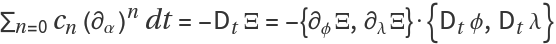. When this condition is satisfied, the exact differential can be integrated to zero around a contour, which implies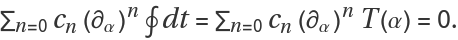The form of the minimal output is an ordinary differential equation constraining period functions T(α).
The proof data described above can be put into an Association:
 PastedInput H Hamiltonian α=H Coordinates {λ,ϕ,α} Tangents {-∂ϕH,∂λH} Time Forms (∂α)ndt,n=0, 1, … ODE Coefficients cn,n=0, 1, … Certificate Function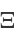Truth Value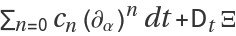The last item with the key "Truth Value" should auto-evaluate to 0 for valid data.
For more details refer to "An Update on the Computational Theory of Hamiltonian Period Functions," especially Chapter 3.
By applying a clever hack, λH can be written as a ratio of λ-polynomials. If it succeeds, the algorithm should proceed rapidly to a solution, whereas if it fails the algorithm stops.
Caveat emptor: This function is effective for a few interesting results but comes with no warranty otherwise!

## Examples

### Basic Examples (3)

Find the period ODE coefficients of a family of elliptic curves in Edwards's normal form:

 In:=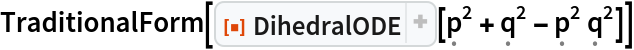Out=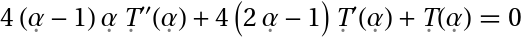Solve the ODE to find the real-valued hypergeometric period function:

 In:=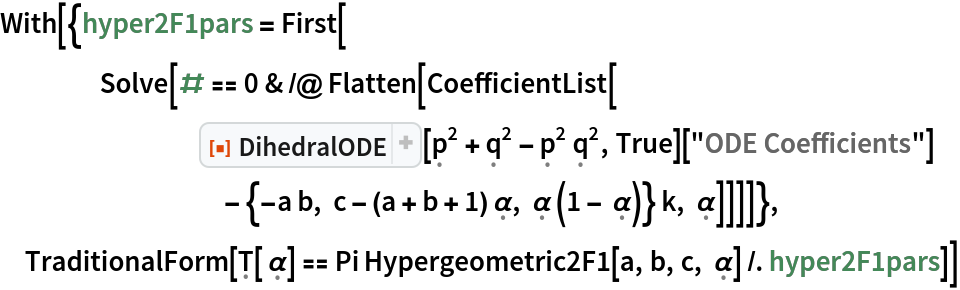Out=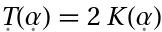Display the proof data in a table:

 In:=Out=### Scope (1)

Despite imperfections, this flexible technique is still quite formidable:

 In:=Out=### Properties and Relations (1)

Proof data is dynamically checkable in a short amount of time:

 In:=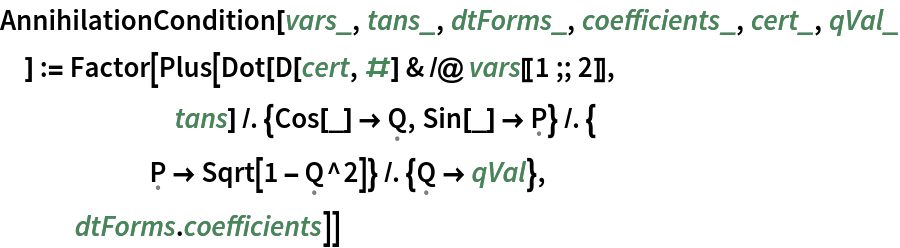In:=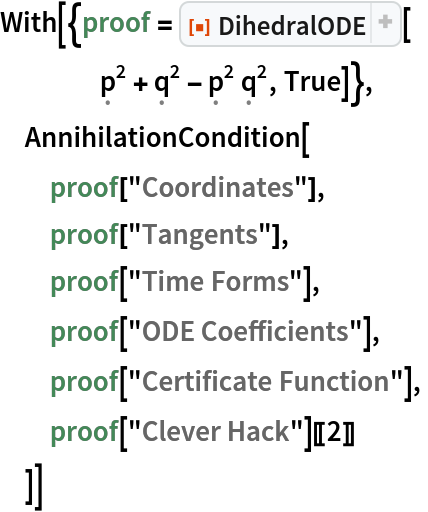Out=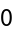### Possible Issues (2)

Hyperelliptic inputs with separable potentials should not be expected to parse:

 In:=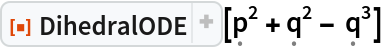Out=If output data becomes weighty, expect a time delay:

 In:=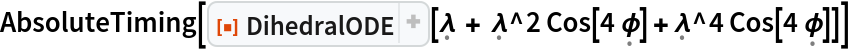Out=### Neat Examples (1)

Discover an apparent genus-degree relation:

 In:=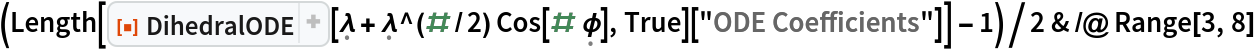Out=